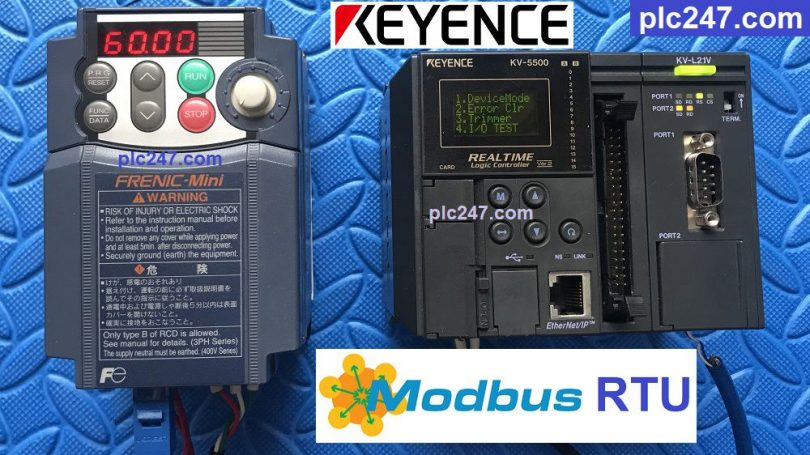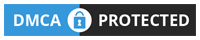# PLC Keyence “Modbus RTU” FUJI FRENIC TutorialWritten by

PLC Keyence is a type of PLC that is new to the market, but its quality is not inferior to any other PLC brand in the world, today plc247.com will guide you to control the FUJI-FRENIC inverter by PLC Keyence.

I will use PLC KV5500 in combination with KV-L21V module to use Modbus-RTU RS485 communication standard

### Connection Diagram

#### Fuji Frenic Mini Inverter Configuration

Modbus-RTU communication parameters to be configured:

+ H30 = 3 (Control via Modbus-RTU)

+ Y01 = 1 (Slave Station Number)

+ Y02 = 0

+ Y03 = 2 (communication error timer)

+ Y04 = 2 (baud rate 9600)

+ Y05 = 0 (use 8bit)

+ Y06 = 1 (Even)

+ Y07 = 1 (Stop bit = 1)

+ Y10 = 0 (Modbus-RTU Selection)

+ Y99 = 0

Below is the Layout table of Hexa addresses corresponding to each parameter range.

##### Register Control Table

Looking at the table above we can see:

+ Frequency Setting Address: S05 = 07Hex + 05 = 705 (Hex)

+ Operation Command Address: S07 = 07Hex + 06 = 706 (Hex)

• Run Forward >>> Set 706 (Hex) = 1
• Run Reverse >>> Set 706 (Hex) = 2
• Stop Run >>> Set 706 (Hex) = 0
• Output Frequency = M (08Hex) + 09 = 809 (Hex)
• Output Voltage = M (08Hex) + 012 = 80C (Hex)

### Keyence KV5500 Programming

KV-L21V Module Setting

+ Write Frequency

+ Motor Control Run

### Detailed Connection Instruction Video

======

Related Documents and Software:

If you have any questions about connecting the Keyence PLC to the FUJI inverter, please comment below the article, I will answer you as soon as I read it.

Best Regards!